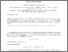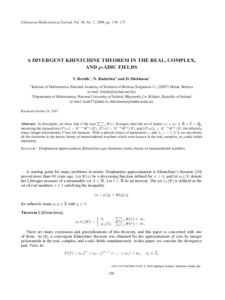# A divergent Khintchine theorem in the real, complex, and p-adic fields

Bernik, Vasili and Budarina, Natalia and Dickinson, Detta (2008) A divergent Khintchine theorem in the real, complex, and p-adic fields. Lithuanian Mathematical Journal, 48 (2). pp. 158-173. ISSN 0363-1672Previewmore...Add this article to your Mendeley library

## Abstract

In this paper, we show that if the sum ∑ r=1 ∞ Ψ(r) diverges, then the set of points (x, z, w) ∈ ℝ × ℂ × ℚp satisfying the inequalities |P(x)|<H−v1Ψλ1(H),|P(z)|<H−v2Ψλ2(H), and |P(w)|p<H−v3Ψλ3(H) for infinitely many integer polynomials P has full measure. With a special choice of parameters v i and λ i , i = 1, 2, 3, we can obtain all the theorems in the metric theory of transcendental numbers which were known in the real, complex, or p-adic fields separately.

Item Type: Article Cite as: Bernik, V., Budarina, N. & Dickinson, D. Lith Math J (2008) 48: 158. https://doi.org/10.1007/s10986-008-9005-9 Diophantine approximation; Khintchine-type theorems metric; theory of transcendental numbers; Faculty of Science and Engineering > Mathematics and Statistics 10111 https://doi.org/10.1007/s10986-008-9005-9 Dr. Detta Dickinson 16 Oct 2018 17:04 Lithuanian Mathematical Journal Springer YesItem control page